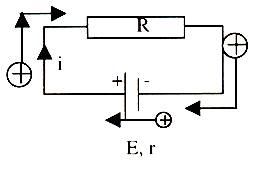# Constant Potential Difference

### Table of Content

Potential difference is an important topic of electric current. Several questions are often asked from this topic in the JEE. It is a simple concept but must be understood properly as it forms the basis of various numerical.

## What exactly is the meaning of Potential?

In simple words, the potential at a point is the potential energy exhibited by a unit charge placed at that point. The term potential should not be confused with potential energy. Potential is the per unit charge of potential energy. The SI unit of measuring potentials is volts.

## Potential Difference

The potential difference between two points A and B is the difference in potentials between A and B. This difference in potentials is also the same as the work done on a unit charge in moving it form B to A. it may also be defined as the electric charge in a circuit between the two points. It is a very important and useful concept of electricity and mostly the concept of potential difference between the points is used rather than the potential at the points.

Let us discuss the concept in detail. We consider the task of moving a positive charge within an electric field from one point say R to another point T. for displacing this charge from one point to another, some work will have to be performed with the help of some external force. When some work is performed on the charge, it brings about a change in its potential energy and raises it to a higher value and this change in potential energy is the same as the amount of work done. This change in potential energy also produces a change in potentials at the points R and T. this difference in potentials is referred to as the electric potential difference. Mathematically, it can be represented as ?

V = VT - VR.

= Work / Charge

= Change in potential energy/ charge

If the potential difference between two points is 2 volts, then one coulomb of charge will gain 2 joules of potential energy when a movement occurs between the two points. Similarly, if the electric potential difference between two locations is 11 volts, then one coulomb of charge gains 11 joules of potential energy when moved from one point to another.

A device used for knowing the potential difference is called a Cell and a combination of these cells is called a Battery. Some internal mechanism exerts forces on the charge carrier of the battery in such a way that the positive charges are driven towards positive terminal from the negative terminal.It should be noted that while in the external circuit positive charges travel from the positive terminal of the battery to the negative terminal, but inside the battery they move from negative to positive terminal. In language of Physics we say that the cell provides electromotive force or emf.

Watch this Video for more reference

The electro-chemical process takes place in the battery which provides electrons at the negative terminal and pulls electrons from the positive terminal as they are necessary for maintaining the current that is being withdrawn. Usually, this reaction utilizes ions and electrons which are representative of positive and negative charge. The reaction takes place at both the electrodes and ceases only when the state of equilibrium is attained. This balance is attained only at a particular voltage. The equilibrium tends to get disturbed when a charge is drawn from some external circuit. Any kind of movement of charge in the external circuit might also result in some kind of internal migration of ions. This balance of ions helps in preserving the level of voltage across terminals and the chemistry also helps in maintaining balance. Hence, the chemical reaction is in accordance with current pulled by the external circuit.

Electric current is basically a flow of charge which in turn is the quantity of electrons. Electrolytes break down into ions, radicals and electrons in solution. The electrodes of the battery are attached to the terminals and hence, the carriers act as free electrons in metals.

A battery also tends to lose voltage as it discharges and finally, it may stop also but only when the chemicals reach some limit of availability. When the reactions cannot supply sufficient charge, the discharging of battery tends to bring about an increase in the internal resistance.

askIITians offers comprehensive study material which covers all the important topics of
IIT JEE Physics. The notes contain numerous solved examples including the ones from previous years. It is important to master the constant difference potential in order to remain competitive in the JEE.

## Related Resources

To read more, Buy study materials of Current Electricity comprising study notes, revision notes, video lectures, previous year solved questions etc. Also browse for more study materials on Physics here.### Course Features

• Video Lectures
• Revision Notes
• Previous Year Papers
• Mind Map
• Study Planner
• NCERT Solutions
• Discussion Forum
• Test paper with Video Solution